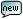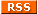Ofer writes: An error in Singh's book Singh, a physicist by training, shows a lack of understanding of Godel's incompleteness theorem. What he writes there about it is utterly wrong.He says that Godel's incompleteness theorem indicates that perhaps 'Fermat's last theorem is undecidable', and hence Wales might be wasting his time because there cannot be a proof.This is not what Godel's incompleteness theorem means at all. Incompleteness does not refer to a single formula (and this is what Fermat's last theorem is - a single quantified formula), rather to an infinite collection of formulas; Incompleteness of such an infinite set (e.g. first order logic) means that there is no finite set of axioms + deductive rules that are on the one hand sound (they only prove correct things), and on the other hand enable us to prove the correctness of all the correct theorems.From what Singh is saying, one may conclude that because Wales proved Fermat's theorem eventually, it turns out that this theory is indeed decidable; but this is simply wrong.  Posted on Thursday, 05 September 2013 at 23:06 GMT [Reply to this] [Permalink]

[Back to the discussion]©1997-2022 by Tal Cohen, all rights reserved. [About]